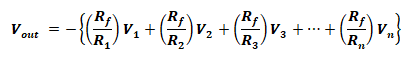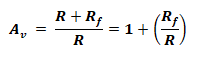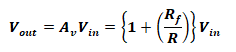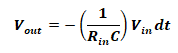# Operational Amplifier (OP-AMP) – Formulas and Equations

## Operational Amplifier (OP-AMP) Formulas### Operational Amplifiers:

#### Inverting Amplifier:The following terms are used in the formulas and equations for Operational Amplifies.

• Rf = Feedback resistor
• Rin = Input Resistor
• Vin ­­­= Input voltage
• Vout = Output voltage
• Av = Voltage Gain

#### Voltage Gain:

The close loop gain of an inverting amplifier is given by;#### Output Voltage:

The output voltage is out of phase with the input voltage that is why it is known as the inverting amplifier.### Summing Amplifier:#### Output Voltage:

The general output of this given circuit above is;#### Inverted Amplified Sum of Input Voltage:

if the input resistors are same, the output is a scaled inverted sum of input voltages,

If R1 = R= R3 = R= R#### Summed Output:

When all the resistors in the above given circuit are same, the output is an inverted sum of input voltages.

If Rf = R1 = R= R3 = R= R;

Vout = – (V+ V2 + V3 +… + Vn)

### Non-Inverting Amplifier:Terms used for Non-Inverting Amplifier formulas and equations.

• Rf = Feedback resistor
• R = Ground Resistor
• Vin ­­­= Input voltage
• Vout = Output voltage
• Av = Voltage Gain

#### Gain Of Amplifier:

The total gain of non-inverting amplifier is;#### Output Voltage:

The output voltage of non-inverting amplifier is in-phase with its input voltage and it’s given by;#### Unity Gain Amplifier / Buffer / Voltage Follower:

If the feedback resistor in removed i.e. Rf = 0, the non-inverting amplifier will become voltage follower/buffer.### Differential Amplifier:Terms used for Differential Amplifier formulas.

• Rf = Feedback resistor
• R= Inverting Input Resistor
• R= Non Inverting Input Resistor
• Rg = Non Inverting ground Resistor
• Va ­­­= Inverting Input voltage
• Vb ­­­= Non Inverting Input voltage
• Vout = Output voltage
• Av = Voltage Gain

#### General Output:

the output voltage of the above given circuits is;#### Scaled Differential Output:

If the resistor Rf = Rg  & R= R, then the output will be scaled difference of the input voltage;#### Unity Gain Difference:

If all the resistors used in the circuit are same i.e. Ra = R= R= R= R, the amplifier will provide output that is the difference of input voltages;

Vout = V– Va

### Differentiator AmplifierThis type of Operational Amplifier provides the output voltage which is directly proportional to the changes in the input voltage. The output voltage is given by;Triangular wave input => Rectangular wave output

Sine wave input => Cosine wave output

#### Integrator AmplifierThis amplifier provides an output voltage which is the integral of the input voltages.Related Formulas and Equations Posts: